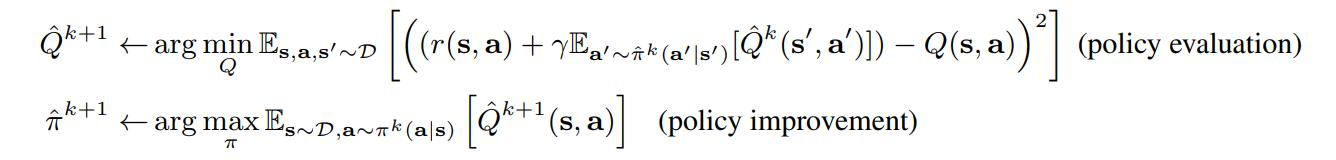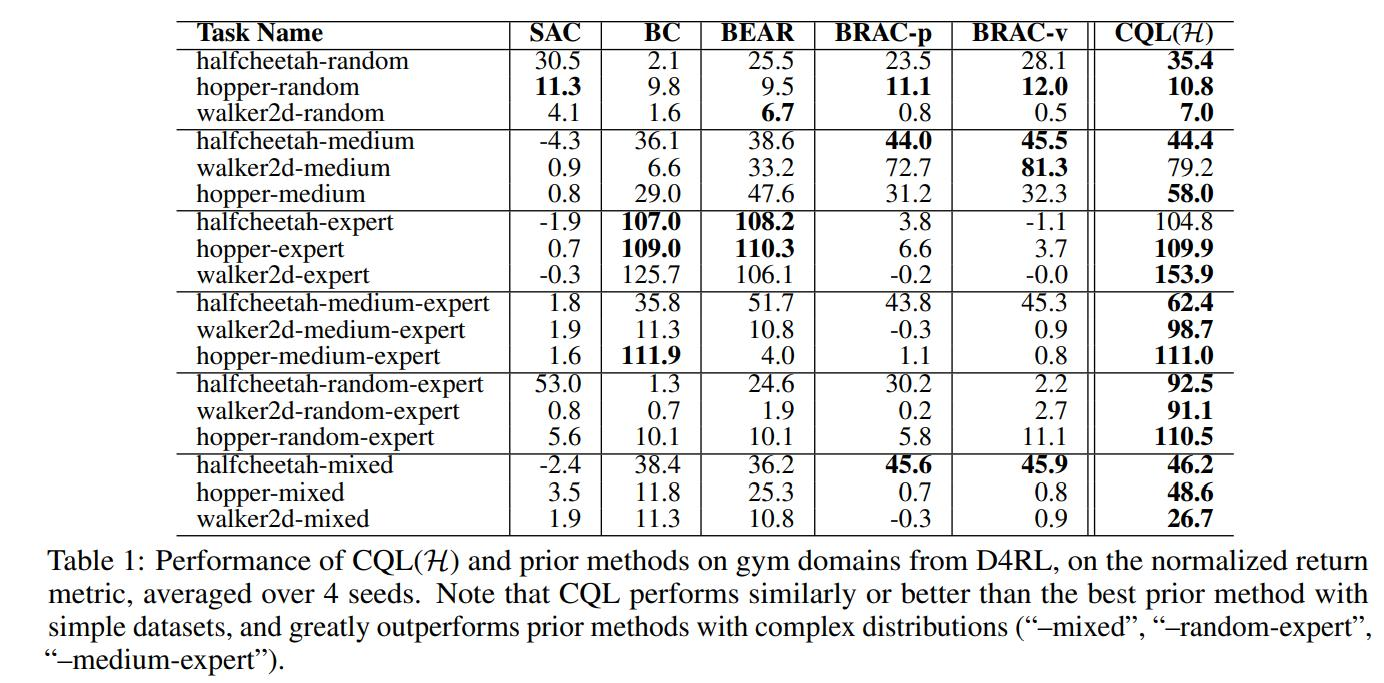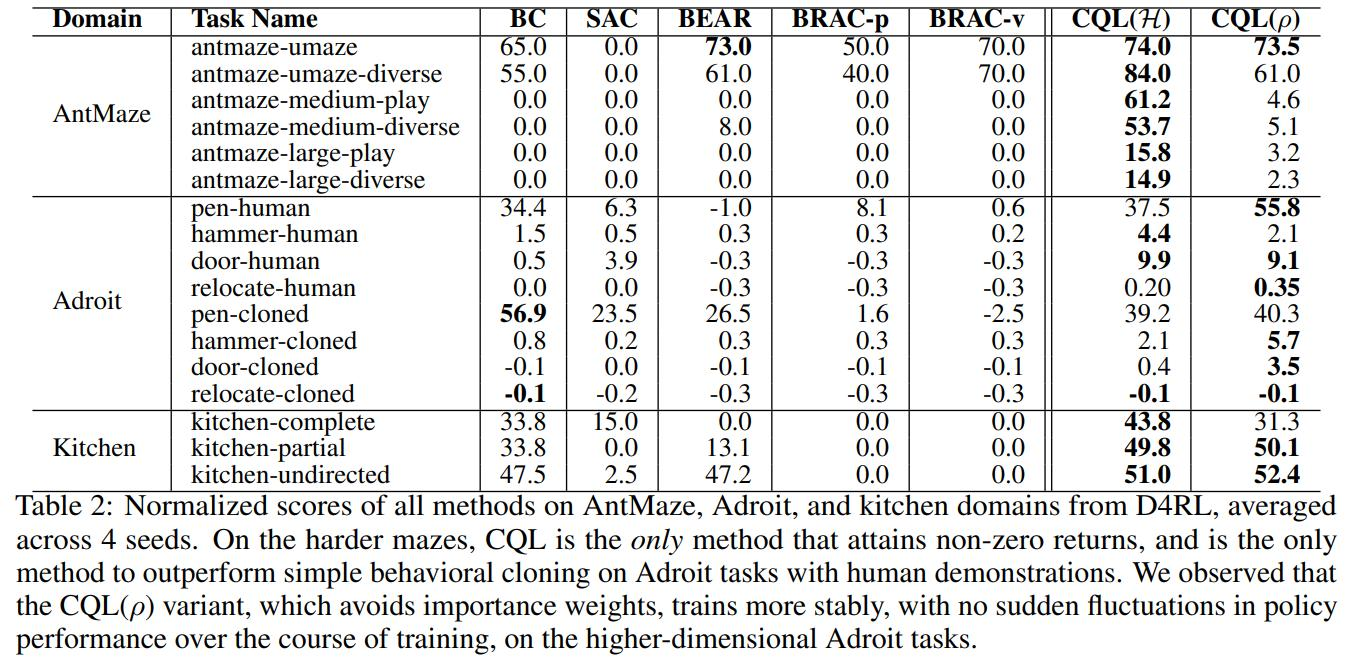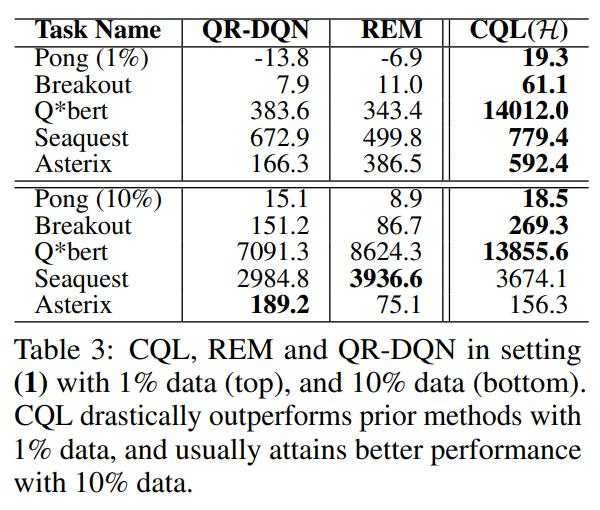# Highlights

• Offline reinforcement learing (RL) algorithms typically suffer from overestimation of the values
• Conservative Q-Learning is introduced to learn a conservative Q-function where the value of a policy under this Q-function lower-bounds its true value
• Works on both discrete and continuous state and action domains

# Introduction

Offline RL aims at learning policies $$\pi$$ that maximize their expected future discounted rewards not by interacting with their environment and receiving rewards but by learning from a static dataset $$\mathcal{D}$$ of transitions $$(s,a,r,s')$$. The dataset $$\mathcal{D}$$ is generated using a behavior policy $$\beta$$ which may be an expert policy, a random policy or a mixture of different policies. Ideally, an agent learning from the dataset would learn the optimal policy $$\pi^*$$ regardless of the behavior policy $$\beta$$.

Because such datasets most often do not include every possible transitions $$(s,a,r,s')$$, the algorithms must rely on standard Temporal-Difference learning methods for learning the Q-function:We can rewrite the policy evaluation equation using the Bellman operator $$\mathcal{B}^\pi$$ as:

$\hat{Q}^{k+1} \gets \argmin_Q \mathbb{E}_{s,a \sim \mathcal{D}} \Big[\big(Q(s,a) - \mathcal{B}^{\pi}Q(s,a)\big)^2\Big],$

where $$\mathcal{B}^{\pi}Q = r + {\gamma}\mathbb{E}_{s' \sim p(s'{\vert}s,a), a' \sim \pi}Q(s',a').$$

However, such methods typically suffer from distributional shift, as the policy learned and used to sample actions $$a'$$ differs from the policy used to generate the dataset. The actions generated by the learning policy may be out-of-distribution from what is present in the dataset and the true Q-value of $$(s', a')$$ may never be encountered. This problem is usually mitigated by actually posing $$a'$$ in $$s'$$ in the online reinforcement learning setting. Adding function approximation errors and more, offline RL methods often learn overestimated Q-values which leads to suboptimal policies.

# Methods

To prevent overestimation of the policy, the authors first propose to modify the update rule of the Q-function to also minimize $$Q$$ under a particular distribution of state-action pairs $$\mu(s,a)$$ while minimizing the Bellman error:

$\hat{Q}^{k+1} \gets \argmin_Q \alpha\big(\mathbb{E}_{s \sim \mathcal{D}, a \sim \mu}Q(s,a)\big) + \frac{1}{2} \mathbb{E}_{s,a \sim \mathcal{D}} \Big[\big(Q(s,a) - \mathcal{B}^{\pi}Q(s,a)\big)^2\Big].$

The authors argue and prove that this lower-bounds the learned Q-function when $$\mu = \pi$$. Then authors then argue and prove that the bound can be tightened by also maximizing the Q-function under the data distribution, leading to the following update:

$\hat{Q}^{k+1}_{\text{CQL}} \gets \argmin_Q \alpha\big(\mathbb{E}_{s \sim \mathcal{D}, a \sim \mu}[Q(s,a)] - \mathbb{E}_{s,a \sim \mathcal{D}}[Q(s,a)]\big) + \frac{1}{2} \mathbb{E}_{s,a \sim \mathcal{D}} \Big[\big(Q(s,a) - \mathcal{B}^{\pi}Q(s,a)\big)^2\Big].$

The conservative Q-function can then be used to perform Q-Learning or train Actor-Critic policies like SAC.

The authors test their CQL update by modifying the SAC algorithm. The SAC algorithm uses a modified version of RL called Maximum-Entropy Reinforcement Learning (MaxEnt-RL), where the objective is to both maximize the expected return and the entropy of the policy, leading to the following objective:

$J(\pi) = \argmax_\pi \sum_{t=0}^T \mathbb{E}_{s,a \sim \pi} \gamma^t r_t(s,a) + \mathcal{H}(\pi(\cdot{\vert}s)).$

They call their MaxEnt version $$CQL(\mathcal{H})$$.

## Data

The authors test their results on the OpenAI Gym suite of robotic control environments as well the Arcade Learning Environment.

# Results# Conclusions

CQL provides a simple modification to the standard Q-Learning or Actor-Critic updates which greatly improve offline reinforcement learning performances.

# Remarks

• The paper is still in its first preprint version on arXiv and so contains several typos, formatting errors and nebulous passages. However, the world of RL moves so fast that its results are already used in several other papers and CQL is already described as state-of-the-art for Offline RL.